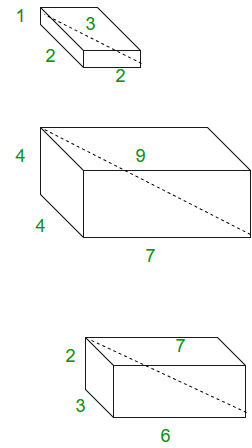• Difficulty Level : Hard
• Last Updated : 22 Jun, 2022

Given four points, check whether they form Pythagorean Quadruple.
It is defined as a tuple of integers a, b, c, d such that. They are basically the solutions of Diophantine Equations. In the geometric interpretation it represents a cuboid with integer side lengths |a|, |b|, |c| and whose space diagonal is |d| .The cuboids sides shown here are examples of pythagorean quadruples.
It is primitive when their greatest common divisor is 1. Every Pythagorean quadruple is an integer multiple of a primitive quadruple. We can generate the set of primitive pythagorean quadruples for which a is odd can be generated by formula :

a = m2 + n2 – p2 – q2
b = 2(mq + np),
c = 2(nq – mp),
d = m2 + n2 + p2 + q2

where m, n, p, q are non-negative integers with greatest common divisor 1 such that m + n + p + q are odd. Thus, all primitive Pythagorean quadruples are characterized by Lebesgue’s identity.

(m2 + n2 + p2 + q2)2 = (2mq + 2nq)2 + 2(nq – mp)2 + (m2 + n2 – p2 – q2)m2 + n2 – p2 – q2

## C++

 `// C++ code to detect Pythagorean Quadruples.``#include ``using` `namespace` `std;` `// function for checking``bool` `pythagorean_quadruple(``int` `a, ``int` `b, ``int` `c,``                                        ``int` `d)``{``    ``int` `sum = a * a + b * b + c * c;``    ``if` `(d * d == sum)``        ``return` `true``;``    ``else``        ``return` `false``;``}` `// Driver Code``int` `main()``{``    ``int` `a = 1, b = 2, c = 2, d = 3;``    ``if` `(pythagorean_quadruple(a, b, c, d))``        ``cout << ``"Yes"` `<< endl;``    ``else``        ``cout << ``"No"` `<< endl;``}`

## Java

 `// Java code to detect Pythagorean Quadruples.``import` `java.io.*;``import` `java.util.*;` `class` `GFG {` `// function for checking``static` `Boolean pythagorean_quadruple(``int` `a, ``int` `b,``                                   ``int` `c, ``int` `d)``{``    ``int` `sum = a * a + b * b + c * c;``    ``if` `(d * d == sum)``        ``return` `true``;``    ``else``        ``return` `false``;``}` `// Driver function``    ``public` `static` `void` `main (String[] args) {``    ``int` `a = ``1``, b = ``2``, c = ``2``, d = ``3``;``    ``if` `(pythagorean_quadruple(a, b, c, d))``        ``System.out.println(``"Yes"``);``    ``else``        ``System.out.println(``"No"` `);``        ` `    ``}``}``// This code is contributed by Gitanjali.`

## Python3

 `# Python  code to detect``# Pythagorean Quadruples.``import` `math` `# function for checking``def` `pythagorean_quadruple(a,b, c, d):` `    ``sum` `=` `a ``*` `a ``+` `b ``*` `b ``+` `c ``*` `c;``    ``if` `(d ``*` `d ``=``=` `sum``):``        ``return` `True``    ``else``:``        ``return` `False` `#driver code``a ``=` `1``b ``=` `2``c ``=` `2``d ``=` `3``if` `(pythagorean_quadruple(a, b, c, d)):``    ``print``(``"Yes"``)``else``:``     ``print``(``"No"` `)` `# This code is contributed``# by Gitanjali.`

## C#

 `// C# code to detect``// Pythagorean Quadruples.``using` `System;` `class` `GFG {` `    ``// function for checking``    ``static` `Boolean pythagorean_quadruple(``int` `a,``                            ``int` `b, ``int` `c, ``int` `d)``    ``{``        ``int` `sum = a * a + b * b + c * c;``        ``if` `(d * d == sum)``            ``return` `true``;``        ``else``            ``return` `false``;``    ``}``    ` `    ``// Driver function``        ``public` `static` `void` `Main () {``            ` `        ``int` `a = 1, b = 2, c = 2, d = 3;``        ` `        ``if` `(pythagorean_quadruple(a, b, c, d))``            ``Console.WriteLine(``"Yes"``);``        ``else``            ``Console.WriteLine(``"No"` `);``            ` `    ``}``}` `// This code is contributed by vt_M.`

## PHP

 ``

## Javascript

 ``

Output:

`Yes`

Time Complexity: O(1)
Auxiliary Space: O(1)

References
Wiki
mathworld

My Personal Notes arrow_drop_up